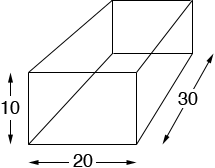SEARCH HOMEMath Central Quandaries & QueriesQuestion from Sara, a student: For my math homework i found the volume of a rectangular solid whose dimensions were in feet and the numbers were 10 by 20 by 30 10 = height 20 = short side base 30 = long side base and now i have to find the surface area of the rectangular solid.... i know how to do this but i can't get the right answer it should be 2,200 ft squared but i keep getting 13,00 feet squaredHi Sara,

Imagine that your rectangular solid is the inside of a cardboard box. There are 6 pieces of cardboard that make up the box, the top and bottom, the front and back and the 2 sides.Cut the box into the 6 pieces. What are the dimensions of each piece? What is the area of each of the 6 pieces? What is the sum of the areas of the 6 pieces?

PennyMath Central is supported by the University of Regina and The Pacific Institute for the Mathematical Sciences.